##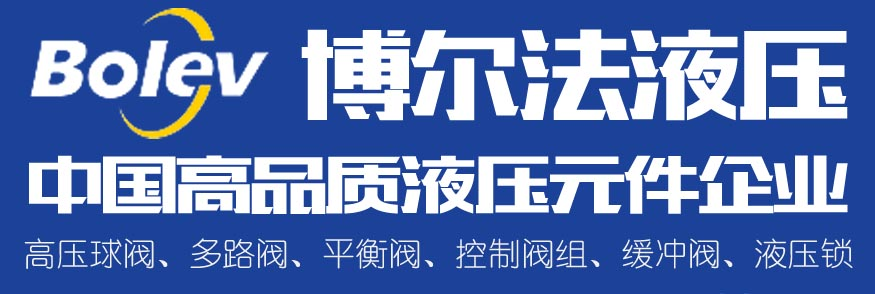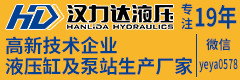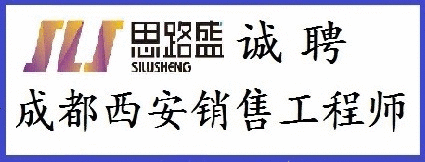多路阀资料下载 标准资料下载 挖掘机资料下载 力士乐资料下载 AMESim资料下载 液压样本下载 液压图书下载 负载敏感资料

# 我会直言不讳但诚实。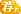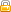[复制链接]• TA的每日心情开心
2019-8-10 23:57
• 签到天数: 62 天

[LV.6]常住居民II发表于 2019-2-3 09:52:33 | 显示全部楼层 |阅读模式
 我以前说过这个。真正的知识并不在于测试书。教科书的作者互相复制，因此每本新书都没有什么新内容。也许有一个不同的解释，人们可能会更好地理解，但书籍中包含的信息基本相同。 缺少的是如何进行闭环控制。问题是即使是教授和许多专业人士也不了解闭环控制。它并不复杂。 下载这个： https://deltamotion.com/peter/Mathcad/Mathcad%20-%20T1C1%20P%20Only%20Laplace.pdf 公式1是控制器功能。在这种情况下，它只是一个比例增益。 公式2是液压执行器的开环传递函数。这与YSX在1982年发表的内容类似。 公式3显示了如何组合控制器和开环传递函数以获​​得闭环传递函数（CLTF） CLTF（s）= Gc（s）* Ga（s）/（1 + Gc（s）* Ga（s）） 哪里： CLTF（S）是使用拉普拉斯变换的s域中的闭环传递函数。 Gc（s）表示s域中控制器的闭环函数。 Ga（s）是s域中液压致动器的开环传递函数。 s是拉普拉斯算子。这是一个频率。 公式4是简化的闭环传递函数。 等式5是闭环传递函数的特征方程。特征方程是闭环传递函数的分母。特征方程确定对控制信号的响应。 等式6a，6b和6c是期望的特征等式。期望的特征方程允许人们在限制内选择期望的响应。 注意，特征方程和期望的特征方程都是三阶多项式。 由于特征和期望的特征方程都具有相等的s ^ 3系数。 s ^ 0，S ^ 1和S ^ 2的系数必须相等。这提供了3个方程来求解3个未知数，如等式7所示。 三个未知数是Kp（比例增益），α（三个闭环极点的实部）和β（三个闭环极点的虚部）。 请注意，解决方案是符号化的。 Kp（比例增益）有一个公式 α的公式（三个闭环极的实部） 存在β的公式（三个闭环极点的虚部）。 请注意，公式都取决于开环系统的参数。这意味着一旦设计了液压系统，试图优化增益的人就不能比公式所预测的更好。 α的单位为1 /次。如果α被反转则它变成时间常数。这是任何仅比例控制器（如步进气缸）的最佳时间常数。 等式13和14提供作为时间函数的位置和速度响应。同样，响应由液压和机械设计决定。这些公式没有任何增益可以使响应更快。最佳响应由开环增益，固有频率和阻尼因子决定。另请注意，r是位置变化量，但误差始终在exp（α* t）处衰减 学习这个。教授需要教这个。没有教授闭环控制。 除非液压人员了解这一点，否则他们将失去使用伺服电机的人。 I am going to be blunt but honest. I have said this before.   The real knowledge does not lie in the test books.  The text book authors copy from each other so there is little new in each new book.  Perhaps there is a different explanation that one may better understand but the books contain basically the same information. What is missing is how to do closed loop control.  The problem is that even the professors and many professionals do not understand closed loop control.   It isn't that complicated. Download this: https://deltamotion.com/peter/Mathcad/Mathcad%20-%20T1C1%20P%20Only%20Laplace.pdf Equation 1 is the controller function.  In this case it is only a proportional gain. Equation 2 is the open loop transfer function for the hydraulic actuator.  This is simiarl to what YSX published in 1982. Equation 3 shows how to combine the controller and open loop transfer functions to get a closed loop transfer function ( CLTF) CLTF(s) = Gc(s)*Ga(s)/(1+Gc(s)*Ga(s)) where: CLTF(S) is the closed loop transfer function in the s domain using Laplace transforms. Gc(s) is the representation of the controller's closed loop function ins the s domain. Ga(s) is the open loop transfer function for the hydraulic actuator in the s domain. s is the Laplace operator.  It is a frequency. Equation 4 is the simplified close loop transfer function. Equation 5 is the characteristic equation of the closed loop transfer function.   The characteristic equation is the denominator of the closed loop transfer function.  The characteristic equation determines the response to a control signal. Equations 6a, 6b, and 6c are the desired characteristic equation.   The desired characteristic equation allows one to chose the desired response within limits. Notice that the characteristic equation and the desired characteristic equation are both third order polynomials. Since both the characteristic and desired characteristic equations both have equal s^3 coefficients.   The coefficents for s^0, S^1, and S^2 must be equal.    This provides 3 equations to solve for 3 unknowns as shown in equation 7. The three unknowns are Kp ( proportional gain ),  alpha ( the real part of the three closed loop poles ) and beta ( the imaginary part of the three closed loop poles ). Notice that the solution is symbolic. There is a formula for Kp ( proportional gain ) There is a formula for α ( the real part of the three closed loop poles ) There is a formula for β  ( imaginary part of the three closed loop poles ). Notice that the formulas all depend on the parameters for the open loop system.  This means that once the hydraulic system is designed,  the person trying to optimize the gains cannot do better than what is predicted by the formulas. α has units of 1/time.   If α is inverted then it becomes a time constant.  This is the optimal time constant for any proportional only controller like a stepper cylinder. Equations 13 and 14 provide the position and velocity response as a function of time.  Again, the response is determined by the hydraulic and mechanical design.  There are no gains in these formulas that will make the response faster.  The optimal response is determined by the open loop gain, natural frequency and damping factor.  Notice also that r is the amount of change in position but the error always decays at exp(α*t) Learn this.  The professors need to teach this.  Close loop control isn't being taught.  What ever the teachers teach you do not believe that is all there is.  There is so much more. Unless hydraulic people learn this they will lose to those using servo motors.
回复 论坛版权
• TA的每日心情慵懒
2016-2-27 10:51
• 签到天数: 8 天

[LV.3]偶尔看看II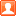发表于 2019-2-4 08:56:44 www.iyeya.cn | 显示全部楼层
 步进液压缸的真正对手是伺服电杆。这主要是功能上原全重叠，连限制也相同。不算成本的话，大部份5吨以下的应用，伺服电杆完勝液压，单是省去液压油这一点，便让我們懂液压的无用武之地！出产步进缸的可考慮把油箱和电控也集成为一体，做成免调试，插电即用的产品来和伺服电缸竞爭。
回复 支持 反对
• TA的每日心情慵懒
2016-2-27 10:51
• 签到天数: 8 天

[LV.3]偶尔看看II发表于 2019-2-5 21:45:54 www.iyeya.cn | 显示全部楼层
 恭喜恭喜。
回复 支持 反对
 本版积分规则 回帖后跳转到最后一页